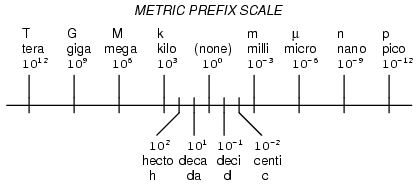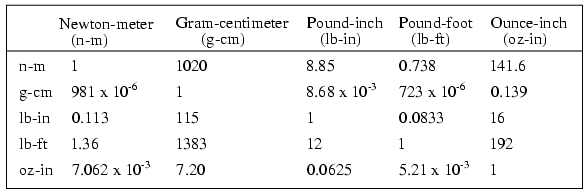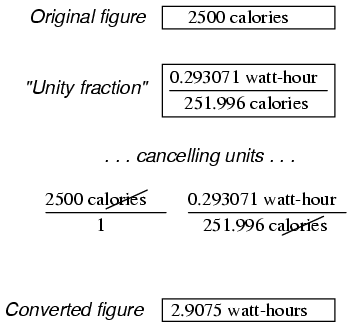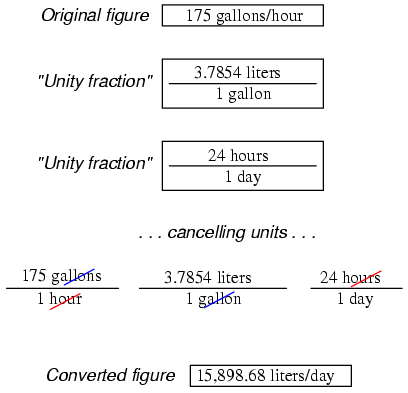# Learning Electronics

Learn to build electronic circuits

# Metric prefixes and unit conversions

• Metric prefixes
• Yotta = 1024 Symbol: Y
• Zetta = 1021 Symbol: Z
• Exa = 1018 Symbol: E
• Peta = 1015 Symbol: P
• Tera = 1012 Symbol: T
• Giga = 109 Symbol: G
• Mega = 106 Symbol: M
• Kilo = 103 Symbol: k
• Hecto = 102 Symbol: h
• Deca = 101 Symbol: da
• Deci = 10-1 Symbol: d
• Centi = 10-2 Symbol: c
• Milli = 10-3 Symbol: m
• Micro = 10-6 Symbol: µ
• Nano = 10-9 Symbol: n
• Pico = 10-12 Symbol: p
• Femto = 10-15 Symbol: f
• Atto = 10-18 Symbol: a
• Zepto = 10-21 Symbol: z
• Yocto = 10-24 Symbol: y• Conversion factors for temperature
• oF = (oC)(9/5) + 32
• oC = (oF - 32)(5/9)
• oR = oF + 459.67
• oK = oC + 273.15

Conversion equivalencies for volume

1 US gallon (gal) = 231.0 cubic inches (in3) = 4 quarts (qt) = 8 pints (pt) = 128 fluid ounces (fl. oz.) = 3.7854 liters (l)
1 Imperial gallon (gal) = 160 fluid ounces (fl. oz.) = 4.546 liters (l)

Conversion equivalencies for distance

1 inch (in) = 2.540000 centimeter (cm)

Conversion equivalencies for velocity

1 mile per hour (mi/h) = 88 feet per minute (ft/m) = 1.46667 feet per second (ft/s) = 1.60934 kilometer per hour (km/h) = 0.44704 meter per second (m/s) = 0.868976 knot (knot -- international)

Conversion equivalencies for weight

1 pound (lb) = 16 ounces (oz) = 0.45359 kilogram (kg)

Conversion equivalencies for force

1 pound-force (lbf) = 4.44822 newton (N)

Acceleration of gravity (free fall), Earth standard

9.806650 meters per second per second (m/s2) = 32.1740 feet per second per second (ft/s2)

Conversion equivalencies for area

1 acre = 43560 square feet (ft2) = 4840 square yards (yd2) = 4046.86 square meters (m2)

Conversion equivalencies for pressure

1 pound per square inch (psi) = 2.03603 inches of mercury (in. Hg) = 27.6807 inches of water (in. W.C.) = 6894.757 pascals (Pa) = 0.0680460 atmospheres (Atm) = 0.0689476 bar (bar)

Conversion equivalencies for energy or work

1 british thermal unit (BTU -- "International Table") = 251.996 calories (cal -- "International Table") = 1055.06 joules (J) = 1055.06 watt-seconds (W-s) = 0.293071 watt-hour (W-hr) = 1.05506 x 1010 ergs (erg) = 778.169 foot-pound-force (ft-lbf)

Conversion equivalencies for power

1 horsepower (hp -- 550 ft-lbf/s) = 745.7 watts (W) = 2544.43 british thermal units per hour (BTU/hr) = 0.0760181 boiler horsepower (hp -- boiler)

Conversion equivalencies for motor torqueLocate the row corresponding to known unit of torque along the left of the table. Multiply by the factor under the column for the desired units. For example, to convert 2 oz-in torque to n-m, locate oz-in row at table left. Locate 7.062 x 10-3 at intersection of desired n-m units column. Multiply 2 oz-in x (7.062 x 10-3 ) = 14.12 x 10-3 n-m.

Converting between units is easy if you have a set of equivalencies to work with. Suppose we wanted to convert an energy quantity of 2500 calories into watt-hours. What we would need to do is find a set of equivalent figures for those units. In our reference here, we see that 251.996 calories is physically equal to 0.293071 watt hour. To convert from calories into watt-hours, we must form a "unity fraction" with these physically equal figures (a fraction composed of different figures and different units, the numerator and denominator being physically equal to one another), placing the desired unit in the numerator and the initial unit in the denominator, and then multiply our initial value of calories by that fraction.

Since both terms of the "unity fraction" are physically equal to one another, the fraction as a whole has a physical value of 1, and so does not change the true value of any figure when multiplied by it. When units are canceled, however, there will be a change in units. For example, 2500 calories multiplied by the unity fraction of (0.293071 w-hr / 251.996 cal) = 2.9075 watt-hours.The "unity fraction" approach to unit conversion may be extended beyond single steps. Suppose we wanted to convert a fluid flow measurement of 175 gallons per hour into liters per day. We have two units to convert here: gallons into liters, and hours into days. Remember that the word "per" in mathematics means "divided by," so our initial figure of 175 gallons per hour means 175 gallons divided by hours. Expressing our original figure as such a fraction, we multiply it by the necessary unity fractions to convert gallons to liters (3.7854 liters = 1 gallon), and hours to days (1 day = 24 hours). The units must be arranged in the unity fraction in such a way that undesired units cancel each other out above and below fraction bars. For this problem it means using a gallons-to-liters unity fraction of (3.7854 liters / 1 gallon) and a hours-to-days unity fraction of (24 hours / 1 day):Our final (converted) answer is 15898.68 liters per day.